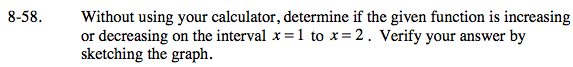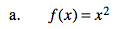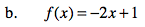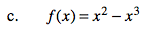### Home > PC > Chapter 8 > Lesson 8.1.4 > Problem8-58

8-58.
1. Without using your calculator, determine if the given function is increasing or decreasing on the interval x = 1 to x = 2. Verify your answer by sketching the graph. Homework Help ✎1. f (x) = x2

2. f (x) = −2x + 1

3. f(x) = x2x3Make a table with the points of interest for each function. If you are having trouble visualizing the points, plot them to determine if the function increases or decreases over the given interval.